# The DC3 Algorithm Made Simple

The DC3 algorithm is a powerful linear time suffix sorting algorithm. If you’re not familiar with suffix sorting, it is the process in which you sort the different parts of a single string. For example banana suffix sorted would be: ``` a ana anana banana na nana ```

Efficient suffix sorting is needed in data compression, and substring matching. Practically speaking, suffix sorting can be used to search for text in an ebook, identifying long repeated sequences of DNA in a genome,  etc.

The most obvious way to suffix sort is to use a n*log(n) comparison algorithm to compare all the suffices of a string. However in the last 20 years, faster linear time suffix sorting algorithms have been developed. These algorithms beat the n*log(n) barrier by avoiding redundant sorting associated with suffix sorting. For example, from the banana suffix sort above, if you have sorted “ana” and “a”,  there is no reason to compare the entire suffices “nana” and “na”. Once you determine that they both start with “n”s you should just use the  result of the “ana” and “a” sort. The DC3 algorithm uses this rationale to create an elegant solution for linear time suffix sorting.  I’ll explain this algorithm by first exploring the process behind it and then by examining a java implementation I created.

## The Process

The easiest way to explain DC3 is to go through an example and explain relevant points along the way. To do this I will sort of the string “yabbadabbado”.

### The Basics

DC3 works by sorting and then merging two different sets of suffices in a given input string, the sample suffices and the non-sample suffices. The sample suffices are used to sort the nonsample suffices and, thus, avoid redundent sorting. Also DC3 only works on integer alphabets. Specifically an alphabet in which the first letter is 0 and the last letter is known. This sounds like a big limitation if we want to sort ASCII or Unicode but it’s actually easy to get around.

### Preparing to sort ”yabbadabbado”

As I mentioned above, we must convert any word we wish to suffix sort with DC3 into an integer alphabet representation. I’ll discuss a method in which we can do this in the Implementation section but for now we can convert ”yabbadabbado” into an integer alphabet by hand. We preserve the alphabetic order in our new representation: “5 1 2 2 1 3 1 2 2 1 3 4″ ( a=1, b=2, d=3, o=4, y=5). We skip 0 because we will use it as a data sentinel to mark the end of the string. Furthermore we will need some extra filler space to aid in sorting later on. For this, we add two more “zeros”. Our string is now “5 1 2 2 1 3 1 2 2 1 3 4 0 0 0″.

### Determining the sample suffices

Before we can begin sorting, we must first determine which indices are part of the “sample”. For DC3, we create a sample from the indices that are not divisible by 3. In mathematical terms, that means the sets B1 and B2 from the formula below where n is the last index in the string.We concatenate B1 and B2 to produce the sample that I call B12, {1, 4, 7, 10, 2, 5, 8, 11}. The diagram makes this partition obvious. Note that I added the set B0.  We don’t need it yet but I thought I’d point it out.
### Radix Sorting B12

After we have created B12, we radix sort the first three letters found at each index to get the sorted order of B12.(Note that when I say “letters” I’m not referring the traditional alphabet but the integers that replaced our ASCII characters) When we finish the radix sort we can rank each index based on the letters we found radix sorting. Heres what the result looks like.Theres a few important things to note. One, the positions 2 and 7 get the same rank, 4, because they point to letter sequences that are equal. Two, the index 11′s letter sequence is 400. The extra zero, the one after the data sentinel, comes from the “filler” we allocated before we started sorting. Had we not allocated filler we would have tried to access unallocated memory during the radix sort and caused an error.

### Evaluating the Results of Radix Sorting the Indices of B12

Unfortunately, since two indices, 2 and 7, have the same rank we must resolve the collision and figure out which index truly has the 4th rank. Fortunately theres a fast linear time suffix sorting algorithm called DC3 that can solve this for us. That’s right, we’ll use recursion. To do this we’ll rename each index with it’s rank. So now we have:Notice that we added 3 zeros to our new array. Again this is useful during radix sorting. We sort this new string with DC3.

### First Level of Recursion

The recursive DC3 call proceeds exactly like I have described so far. We determine the sample suffices, and we radix sort them. I’ll skip explaining these steps but show them in the diagram below.
This time we finish radix sorting the input and find that the sort is unique. No indices are given the same rank. We can move on to sorting the non sample suffices for the string: “1  2  4  6  4  5  3  7  0″.

### Sorting the Nonsample Suffices (Still in the First Recursive Call)

To sort the non sample suffices, set B0, we will use the ranks found during the last radix sort and the “non-sample” letters,  the letters pointed to by the values in B0. We use this information to create two letter pairs that can be compared against one another. The most significant letter in a pair is the ”non-sample” letter. The second most significant letter in a pair is the rank found at the index of the ”non-sample” letter plus one.  A diagram can help explain:
Notice that we made the ranks of the filler positions  0. This helps us avoid accessing unallocated memory and allows us to sort the non sample suffices correctly. Next we radix sort the pairs:
Radix sorting the pairs gives us the sorted order of the non sample suffices, 0 6 3. One thing to note here is the importance of unique ranks. When we sorted the sample suffices we discovered that each index in the sample had a unique rank. Had this not been the case we could not have sorted B0 because there is a possibility for ties. For DC3 to work, there cannot be ties when sorting the non sample suffices.

### Merging B0 and B12 (Still in the First Recursive Call)

Now that we have sorted the sample and non sample suffices we can merge the two to come up the sorted indices of the string “1  2  4  6  4  5  3  7  0″. To do the merge we will use special rules for the two possible cases, comparing a member of B0 against a member of B1 and comparing a member of B0 against a member of B2.

B0 vs B1

Let bo and b1 be the indices we are trying to compare from sets B0 and B1 respectively. In this case, we first compare the letters at the indices b0 and b1. If there is a tie, we will compare the ranks located at b0+1 and b1+1.

B0 vs B2

Let bo and b2 be the indices we are trying to compare from sets B0 and B2 respectively. In this case, we first compare the letters at the indices b0 and b2. If there is a tie, we will compare the letters at indices b0+1 and b2+1. If there is still a tie we compare the the ranks located at b0+2 and b0+2.

It’s important to note that there can never be a tie during the comparisons of this stage. This is impossible because the final stage of each comparison employs comparing two different ranks. We know each rank is unique because the “sample” sorting phase either discovered that we had uniquely sorted the sample indices or called upon DC3 to create a unique ranks.

Now lets look at these rules in practice by getting back to our example. Below is a detailed look at how the merge proceeds.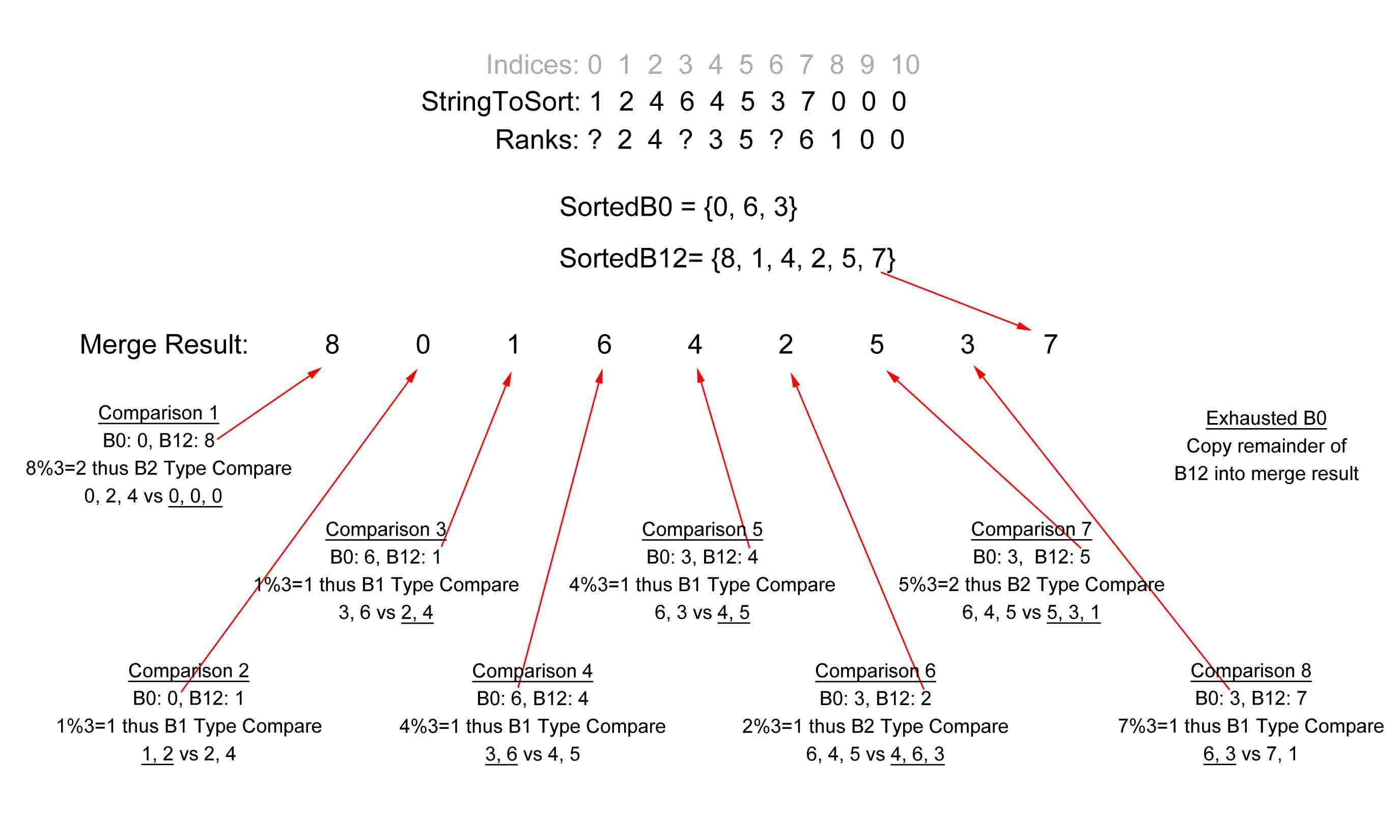Finally we are done with the recursive step of this problem! Lets take a look at where we stepped into recursion and figure out how to interpret the result.

### Coming back from Recursion

In the sort of “yabbadabbado” we stepped into recursion because we couldn’t uniquely sort the sample suffices B12: {1, 4, 7, 10, 2, 5, 8, 11}. Now we have have the sorted order of these indices after being replaced with their ranks. We can use this sort to uniquely sort B12. This part is confusing so reread the next paragraph if you don’t understand at first.

The rank replacement allowed us to sort the “value” at each index. This value was based on the first three letters found at the index. The recursive step sorts these values further and in the process finds a unique sort. By sorting only the values we can effectively compare more than 3 letters at a time. For example in the first level of recursion you compare the values of  the values generated during the first (non recursive radix sort). The values at the first level of recursion would then be based on 6 different letters. Another important thing to note is that we find a unique sort relatively quickly. Each time we step into recursion we reduce the input string’s size by roughly one third. An input string of any size is quickly reduced to small problem. This allows DC3 to run in linear time. I’ll look at the running time in detail at the end of the Process section.

Getting back to our example, we can use the return value from the recursive step, {8, 0, 1, 6, 4, 2, 5, 3, 7}, to create a unique sort for B12. Lets call the returned array recReturn, for “recursive return”. We can sort B12 by traversing recReturn  from 1 to recReturn.length-1 and using the value at each index of recReturn to index into B12. The value we find at B12 is placed into the next value of our growing sorted B12 array. Theres a lot of indexing into arrays and a diagram can help clarify:Notice that we skipped the first index of recReturn. We did this because it is always the “mark” zero that we added. We don’t need it to create sortedB12.

To finish interpreting recReturn we need to create ranks for each index in B12. Luckily this step is easy. We traverse sortedB12 and use the value found at each index to index into our ranks array and update value found with a simple counter.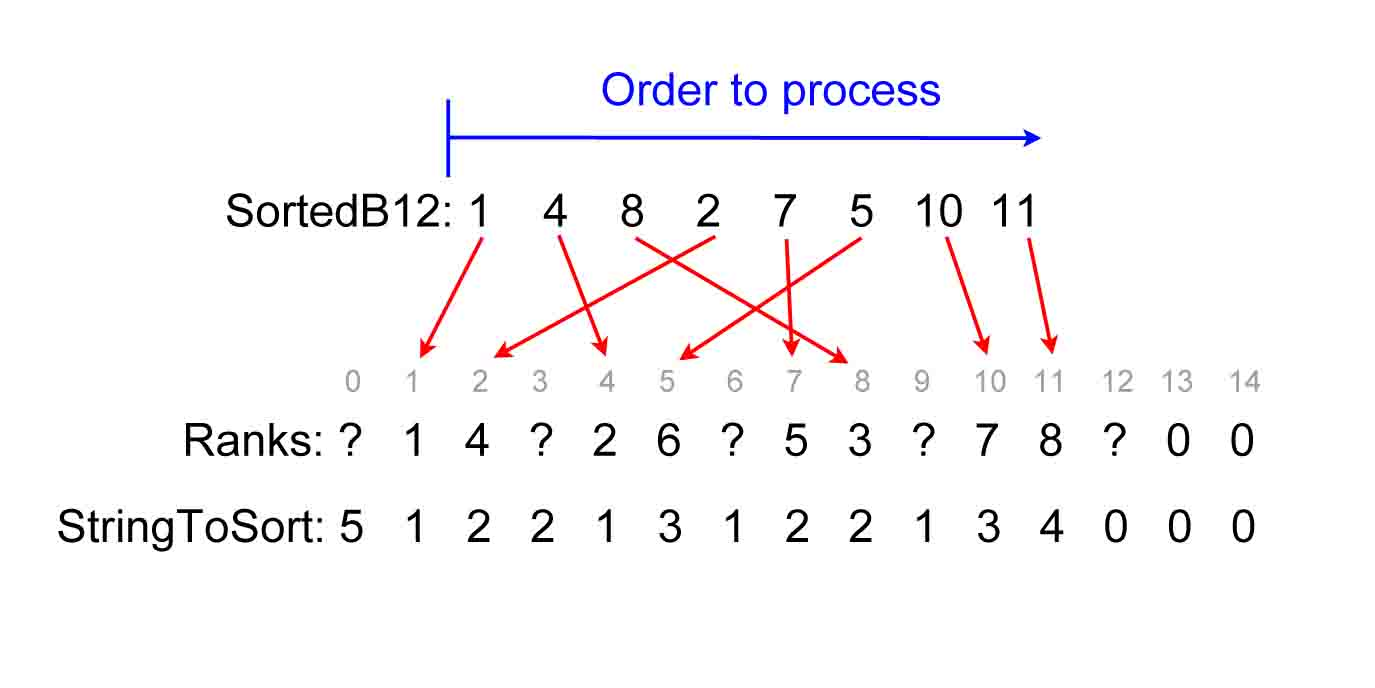Now that we have sortedB12 and found unique ranks we can sort the non sample suffices. This step proceeds just like it did when we sorted the non sample suffices in the recursive call. I will not explain these steps again but will show the results with a diagram.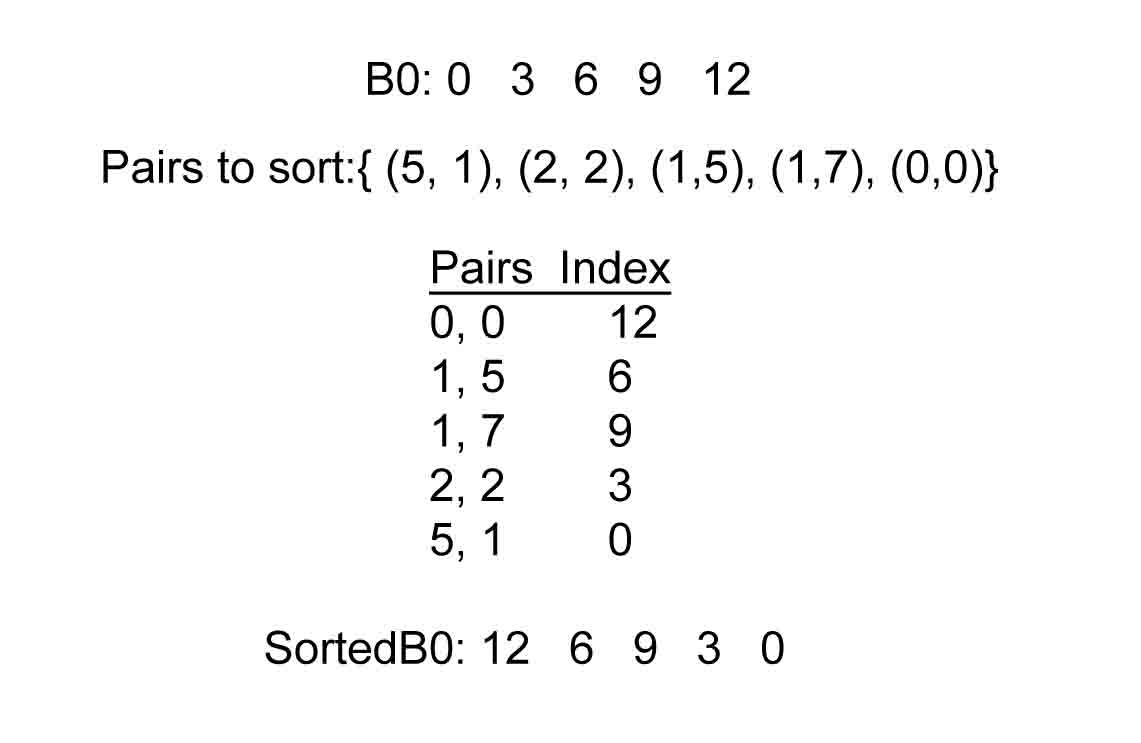Now we merge sortedB0 and sortedB12 just like we did previously.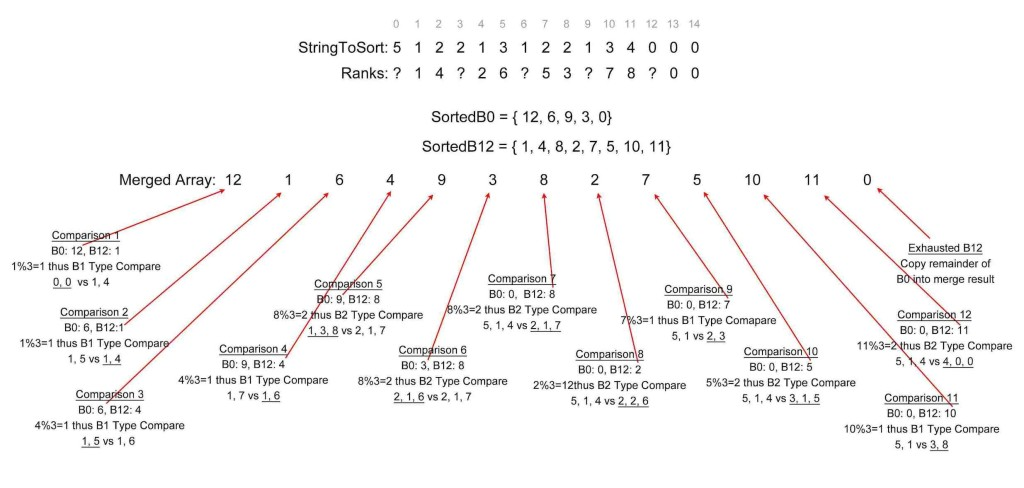Finally we have our output, the int array: { 12, 1, 6, 4, 9, 3, 8, 2, 7, 5, 10, 11, 0}

Heres the suffices that each index corresponds in the original string, “yabbadabbado”.

``` 12: Nothing, this was the mark index 1:  abbadabbado 6:  abbado 4:  adabbado 9:  ado 3:  badabbado 8:  bado 2:  bbadabbado 7:  bbado 5:  dabbado 10: do 11: o 12: yabbadabbado```

As you can see, the DC3 algorithm has sorted our input string! Before jumping to my java implementation of DC3 I’d like to look at the running time of the algorithm.

### DC3 Running Time

It’s pretty easy to see that the individual steps of DC3 run in at most linear time. Even the most intense sorting, the radix sort in the “sample” sort phase, sorts in O(n) time. It radix sorts the first 3 letters of only two thirds of the suffices: f(n) = 3(2n/3)= 2n -> O(n). Note that radix sorting takes time equivalent to the number of strings to sort times the number of characters to sort, or:  f(n) = n*numLetters.

The real question is how many layers of recursion are there?  Every layer of recursion only sorts two thirds of the input string. This gives us the following recursive function: T(n) = T(2n/3) + O(n) which yields a big O time of O(n). This function shows that even large input strings are quickly reduced to small sizes.  To see this more explicitly , you can use the following formula to approximate the the size of the string being sorted at given layer of recursion: recSize=origSize*(2/3)^depthOfRecursion, where recSize is the size of the input string at that depth of recursion, origSize is the size of the original string and depthOfRecursion is the depth of recursion.

Update: I have built the algorithm and it is fast as promised! I’m working on the implementation write up and will post the source code soon when I’m done with the write up.  I used the DC3 sort to speed up the Burrows Wheeler Transform. I ran two different versions of Burrows Wheeler, BWTArraysSort and BWTDC3Sort, to transform pipi.text(the first 500,000 digits of pi repeated twice). BWTArraysSort uses the java Arrays.sort(String) method which runs in n*log(n) time. BWTDC3Sort uses a fast linear time algorithm. Check out the results. I’ll be posting code and explanation of how it works soon